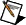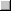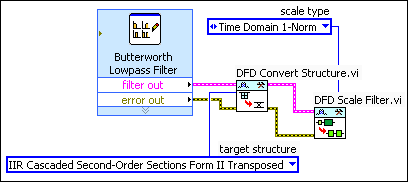# Designing a Fixed-Point Filter Part 2: Scaling Filter Coefficients (Digital Filter Design Toolkit)

In Part 1 of this tutorial, you selected a filter structure for the reference floating-point filter. In Part 2 of this tutorial, you scale the coefficients of the filter.

Every filter structure contains accumulators, which might use different data ranges. In this situation, scaling the coefficients of the reference floating-point filter modifies the accumulators to use the same data range and can help you obtain a better filtering result.Note  If you did not complete Part 1 of this tutorial, refer to labview\examples\Digital Filter Design\Getting Started\Tutorials\Designing a Fixed-Point Filter\Designing a Fixed-Point Filter Part 1.vi for a completed version of the digital filter from that part.

Complete the following steps to scale the coefficients of the reference floating-point filter by using the DFD Scale Filter VI.

1. Place the DFD Scale Filter VI on the block diagram.PlaceFind

2. Wire the filter out and error out outputs of the DFD Convert Structure VI to the filter in and error in inputs of the DFD Scale Filter VI.
3. Right-click the scale type input of the DFD Scale Filter VI and select Create»Constant from the shortcut menu.

A block diagram constant appears above the DFD Scale Filter VI. This constant represents the scale type that this VI uses to scale the filter coefficients.
4. Click the scale type constant to select the scale type this VI uses to scale the filter coefficients.Note  This tutorial uses Time Domain 1-Norm, the default scale type.

The block diagram now resembles the following figure.5. Select File»Save to save the VI.

After you scale the filter coefficients, you can quantize the coefficients of the reference floating-point filter in Part 3 of this tutorial.Note  Refer to labview\examples\Digital Filter Design\Getting Started\Tutorials\Designing a Fixed-Point Filter\Designing a Fixed-Point Filter Part 2.vi for a completed version of the digital filter from this part of the tutorial.